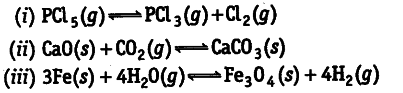# Does the number of moles of reaction products

Does the number of moles of reaction products
increase, decrease or remain same when each of the following equilibria is subjected to a decrease in
pressure by increasing the volume?According to Le-Chatelier’s principle, on decreasing pressure equilibrium shifts in that direction where pressure increases (i.e., number of moles in gaseous state are more). Therefore, number of moles of reaction products in (a) increases (ii) decreases (iii) remains the same.